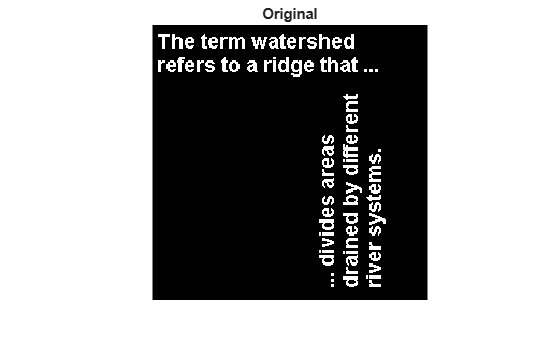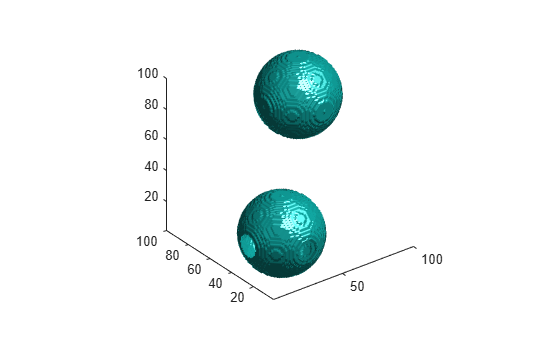imdilate

Description

example

J = imdilate(I,SE) dilates the grayscale, binary, or packed binary image I using the structuring element SE.

J = imdilate(I,nhood) dilates the image I, where nhood is a matrix of 0s and 1s that specifies the structuring element neighborhood.

This syntax is equivalent to imdilate(I,strel(nhood)).

J = imdilate(___,packopt) specifies whether I is a packed binary image.

example

J = imdilate(___,shape) specifies the size of the output image.

Examples

collapse all

Read a binary image into the workspace.

Create a vertical line shaped structuring element.

se = strel('line',11,90);

Dilate the image with a vertical line structuring element and compare the results.

BW2 = imdilate(BW,se);
imshow(BW), title('Original')figure, imshow(BW2), title('Dilated')Read a grayscale image into the workspace.

Create a nonflat ball-shaped structuring element.

se = offsetstrel('ball',5,5);

Dilate the image.

dilatedI = imdilate(originalI,se);

Display the original image and the dilated image.

imshowpair(originalI,dilatedI,'montage')Create two flat, line-shaped structuring elements, one at 0 degrees and the other at 90 degrees.

se1 = strel('line',3,0)
se1 =
strel is a line shaped structuring element with properties:

Neighborhood: [1 1 1]
Dimensionality: 2

se2 = strel('line',3,90)
se2 =
strel is a line shaped structuring element with properties:

Neighborhood: [3x1 logical]
Dimensionality: 2

Dilate the scalar value 1 with both structuring elements in sequence, using the 'full' option.

composition = imdilate(1,[se1 se2],'full')
composition = 3×3

1     1     1
1     1     1
1     1     1

Create a logical 3D volume with two points.

BW = false(100,100,100);
BW(25,25,25) = true;
BW(75,75,75) = true;

Dilate the 3D volume using a spherical structuring element.

se = strel('sphere',25);
dilatedBW = imdilate(BW,se);

Visualize the dilated image volume.

figure
isosurface(dilatedBW, 0.5)Input Arguments

collapse all

Input image, specified as a grayscale image, binary image, or packed binary image of any dimension.

Data Types: single | double | int8 | int16 | int32 | uint8 | uint16 | uint32 | logical

Structuring element, specified as a scalar strel object or offsetstrel object. SE can also be an array of strel object or offsetstrel objects, in which case imdilate performs multiple dilations of the input image, using each structuring element in succession.

imdilate performs grayscale dilation for all images except images of data type logical. In this case, the structuring element must be flat and imdilate performs binary dilation.

Structuring element neighborhood, specified as a matrix of 0s and 1s.

Example: [0 1 0; 1 1 1; 0 1 0]

Indicator of packed binary image, specified as one of the following.

Value

Description

'notpacked'

I is treated as a normal array.

'ispacked'

I is treated as a packed binary image as produced by bwpack. I must be a 2-D uint32 array and SE must be a flat 2-D structuring element. The value of shape must be 'same'.

Data Types: char | string

Size of the output image, specified as one of the following.

Value

Description

'same'

The output image is the same size as the input image. If the value of packopt is 'ispacked', then shape must be 'same'.

'full'

Compute the full dilation.

Data Types: char | string

Output Arguments

collapse all

Dilated image, returned as a grayscale image, binary image, or packed binary image. If the input image I is packed binary, then J is also packed binary. J has the same data type as I.

collapse all

Binary Dilation

The binary dilation of A by B, denoted AB, is defined as the set operation:

$A\oplus B=\left\{z|{\left(\stackrel{^}{B}\right)}_{z}\cap A\ne \varnothing \right\},$

where $\stackrel{^}{B}$ is the reflection of the structuring element B. In other words, it is the set of pixel locations z, where the reflected structuring element overlaps with foreground pixels in A when translated to z. Note that some applications use a definition of dilation in which the structuring element is not reflected.

Grayscale Dilation

In the general form of grayscale dilation, the structuring element has a height. The grayscale dilation of A(x, y) by B(x, y) is defined as:

$\left(A\oplus B\right)\left(x,y\right)=\mathrm{max}\left\{A\left(x-{x}^{\prime },y-{y}^{\prime }\right)+B\left({x}^{\prime },{y}^{\prime }\right)|\left({x}^{\prime },{y}^{\prime }\right)\in {D}_{B}\right\},$

where DB is the domain of the structuring element B and A(x, y) is assumed to be –∞ outside the domain of the image. Note that some applications define grayscale dilation using an equation with A(x + x′, y + y′) instead of A(xx′, yy′).

To create a structuring element with nonzero height values, use the syntax strel(nhood,height), where height gives the height values and nhood corresponds to the structuring element domain, DB.

Most commonly, grayscale dilation is performed with a flat structuring element (B(x,y) = 0). Grayscale dilation using such a structuring element is equivalent to a local-maximum operator:

$\left(A\oplus B\right)\left(x,y\right)=\mathrm{max}\left\{A\left(x-{x}^{\prime },y-{y}^{\prime }\right)|\left({x}^{\prime },{y}^{\prime }\right)\in {D}_{B}\right\}.$

All of the strel syntaxes except for strel(nhood,height), strel('arbitrary',nhood,height), and strel('ball',___) produce flat structuring elements.

Tips

• If the dimensionality of the image I is greater than the dimensionality of the structuring element, then the imdilate function applies the same morphological dilation to all planes along the higher dimensions.

You can use this behavior to perform morphological dilation on RGB images. Specify a 2-D structuring element for RGB images to operate on each color channel separately.

• When you specify a structuring element neighborhood, imdilate determines the center element of nhood by floor((size(nhood)+1)/2).

• imdilate automatically takes advantage of the decomposition of a structuring element object (if it exists). Also, when performing binary dilation with a structuring element object that has a decomposition, imdilate automatically uses binary image packing to speed up the dilation .

 Gonzalez, Rafael C., Richard E. Woods, and Steven L. Eddins. Digital Image Processing Using MATLAB. Third edition. Knoxville: Gatesmark Publishing, 2020.

 Haralick, Robert M., and Linda G. Shapiro. Computer and Robot Vision. 1st ed. USA: Addison-Wesley Longman Publishing Co., Inc., 1992, pp. 158-205.

 Boomgaard, Rein van den, and Richard van Balen. “Methods for Fast Morphological Image Transforms Using Bitmapped Binary Images.” CVGIP: Graphical Models and Image Processing 54, no. 3 (May 1, 1992): 252–58. https://doi.org/10.1016/1049-9652(92)90055-3.